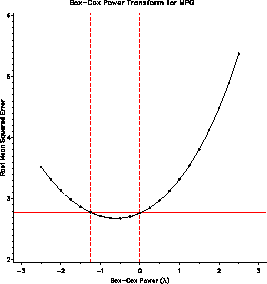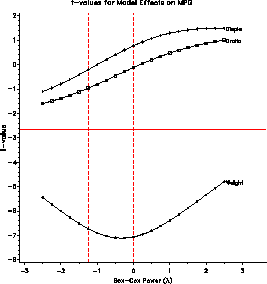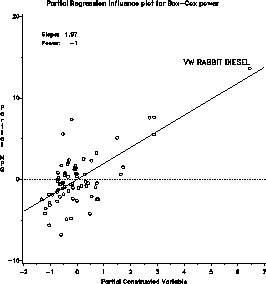boxcox Power transformations by Box-Cox method boxcox

# SAS Macro Programs: boxcox

\$Version: 1.4 (05 Sep 2006)
Michael Friendly
York University

## The boxcox macro (get boxcox.sas)

### Power transformations by Box-Cox method

The boxcox macro finds maximum likelihood power (or folded power) transformations of the response variable in a regression model by the Box-Cox method. The program provides graphic displays of the maximum likelihood solution, t-values for model effects, and the influence of observations on choice of power. The program can produce printer plots or high-resolution versions of any of these plots. The optimal transformation of the response variable is returned in an output dataset.

For a positive response variable, y > 0, the family of monotone power transformations with power p is
 y(p) = (yp - 1) / p p != 0 log (y) p = 0
If the response contains 0 or negative values, use the ADD= parameter to assure that y+&ADD is strictly positive.

If the response variable is bounded on a closed interval, [0, b], the FOLD= parameter may be used to obtain analogous folded-power transformations. For example, use FOLD=100 when the response variable is a percentage on the interval [0, 100].

### Method

The program uses transforms the response to all powers from the LOPOWER= value to the HIPOWER= value, and fits a regression model for each, extracting values to an output dataset from which the plots are drawn.

The influence plot also implements a score test for the power transformation due to Atkinson, which provides an alternative estimate of the power transformation, based on power = 1 - slope of the fitted line in the partial regression plot for a constructed variable.

## Usage

boxcox is a macro program. A value must be supplied for the RESP= parameter.

The arguments may be listed within parentheses in any order, separated by commas. For example:

```   %boxcox(resp=responsevariable, model=predictors, ..., )
```

### Parameters

RESP=
The name of the response variable for analysis.
MODEL=
A blank-separated list of the independent variables in the regression, i.e., the terms on the right side of the = sign in the MODEL statement for PROC REG. The MODEL= terms may be empty to obtain a transformation of a response on its own.
DATA=_LAST_
The name of the data set holding the response and predictor variables. (Default: most recently created)
ID=
The name of an ID variable for observations, used in labeling the influence plot. (Default: ID=_N_)
FOLD=0
Upper bound for the response variable. If FOLD>0 is specified, folded power transformations are computed. E.g., for a response which is a proprotion, specify FOLD=1; for a percentage, specify FOLD=100.
OUT=_DATA_
The name of an output dataset to contain the transformed response. This dataset contains all original variables, with the transformed response replacing the original variable.
OUTPLOT=_PLOT_
The name of the output data set containing _RMSE_, and t-values for each effect in the model, with one observation for each power value tried.
PPLOT=RMSE EFFECT INFL
Which printer plots should be produced? One or more of RMSE, EFFECT, and INFL, or NONE.
GPLOT=RMSE EFFECT INFL
Which high-resolution (PROC GPLOT) plots should be produced? One or more of RMSE, EFFECT, and INFL, or NONE.
LOPOWER=-2
low value for power
HIPOWER=2
high value for power
NPOWER=21
number of power values in the interval LOPOWER to HIPOWER
CONF=.95
confidence coefficient for the confidence interval for the power.

### Example

The example finds power transformations for the MPG variable in the auto dataset, using Weight, Displacement and Gear Ratio as predictors.
```%include data(auto);
%include macros(boxcox);     * or, store in autocall library;
%boxcox(data=auto,
resp=MPG,
model=Weight Displa Gratio,
id=model,
gplot=RMSE EFFECT INFL,
lopower=-2.5, hipower=2.5, conf=.99);
```
The plot of RMSE vs. lambda (power) indicates power = -1 / sqrt(MPG) as the maximum likelihood estimate, but power = -1 / MPG == gallons/mile is within the confidence interval.

The EFFECT plot indicates that the significance of partial t-test are unaffected by the choice of power. The influence plot indicates that the VW Diesel has a large leverage, but is not influential in determining the choice of power.### See also

boxglm Power transformations by Box-Cox method for GLM
boxtid Power transformations by Box-Tidwell method
nqplot Normal QQ plot
outlier Robust multivariate outlier detection
resline Resistant line for bivariate data
stars Diagnostic plots for transformations to symmetry